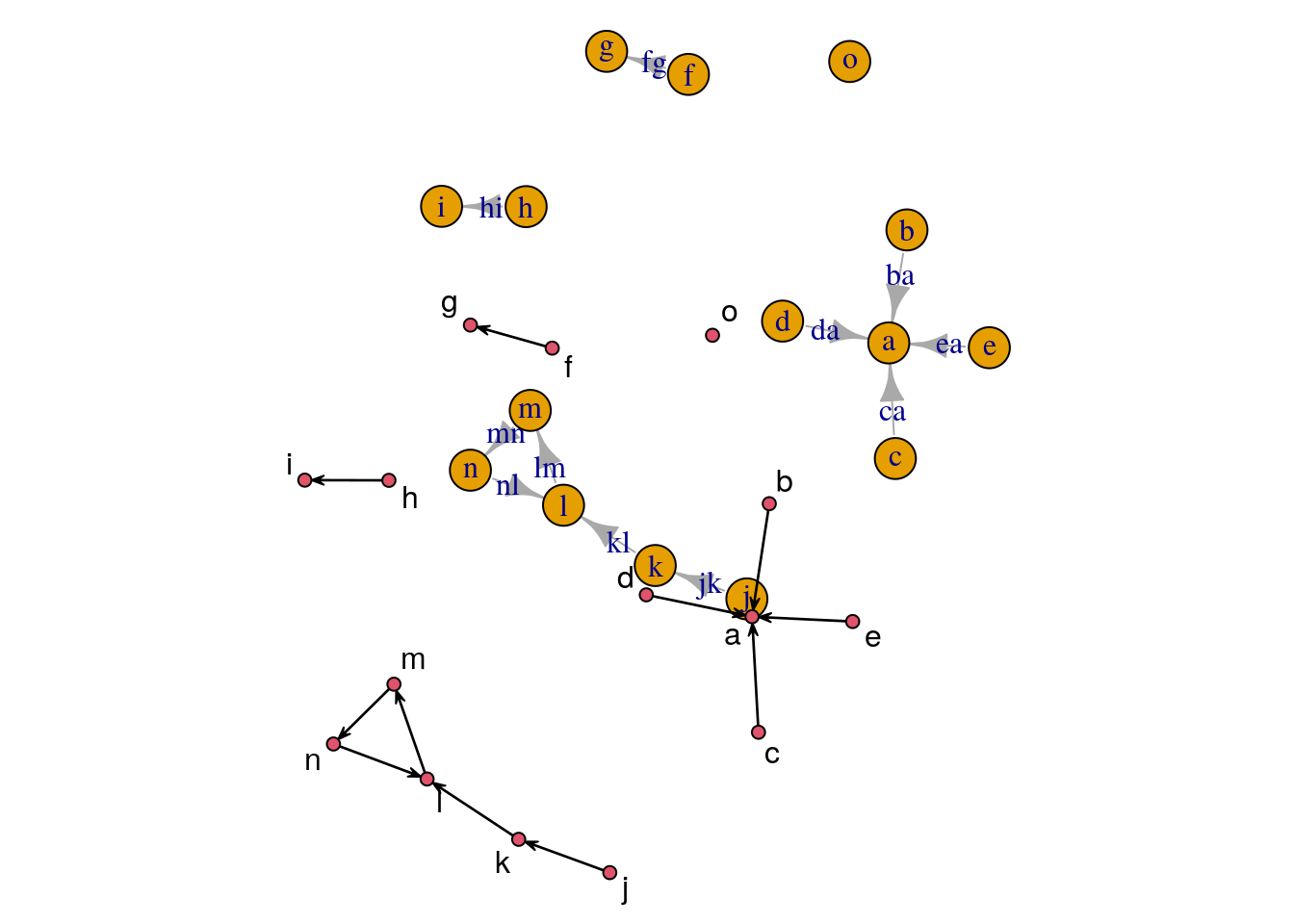After some e-mails I decided to put together a bit more detailed instruction how to install the ‘intergraph’ package together with the namespaced version of the ‘network’ package. See here.

There seemed to be some problems with downloading the namespaced ‘network’ from my webstite (thanks Neil!). File permissions were set incorrectly. I fixed that and everything should be OK now.

Because it’s Friday, another example of using ‘intergraph’. This time plot a network using both ‘igraph’ and ‘network’ functionality on the same plotting device. Here is the result:

``requireNamespace("igraph")``
``## Loading required namespace: igraph``
``library(network)``
``````## network: Classes for Relational Data
## Version 1.16.1 created on 2020-10-06.
## copyright (c) 2005, Carter T. Butts, University of California-Irvine
##                     Mark S. Handcock, University of California -- Los Angeles
##                     David R. Hunter, Penn State University
##                     Martina Morris, University of Washington
##                     Skye Bender-deMoll, University of Washington
##  For citation information, type citation("network").
##  Type help("network-package") to get started.``````
``````library(intergraph)

# rescale 'x' to [-1; 1]
res <- function(x) {
a <- -1 - (2*min(x))/(max(x)-min(x))
b <- 2 / (max(x) - min(x))
a + b*x
}
op <- par(mar=rep(0.5,4))
net <- asNetwork(exIgraph) # using 'intergraph'
set.seed(12345)
k <- apply(igraph::layout.fruchterman.reingold(exIgraph), 2, res)
lx <- c(-0.5, -1)
k2 <- sweep(k, 2, lx, "+")
plot(exIgraph, layout=k, rescale=FALSE, xlim=range(rbind(k, k2)[,1]),
ylim=range(rbind(k, k2)[,2]) )
plot(net, coord=k2, displaylabels=TRUE, label=net %v% "label", new=FALSE)````````par(op)``

And here is the code to produce it. `lx` in line 13  is “Deus Ex Machina”, or rather “Deus Ex locator()” 😉